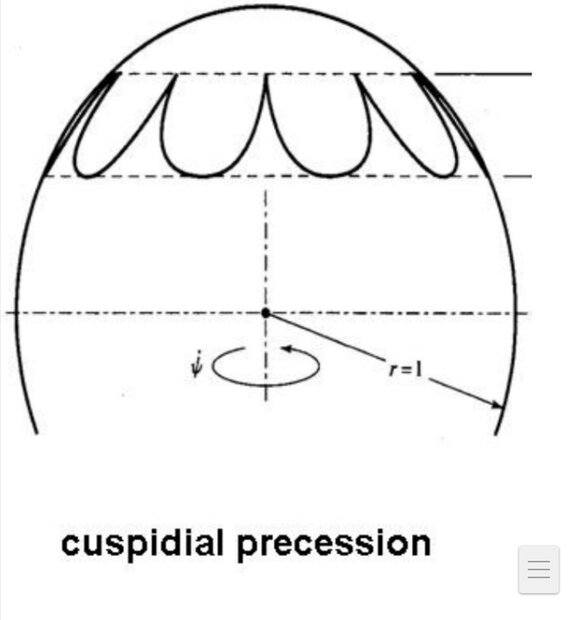# Locus if velocity component is zero

• B
KashmirFigure shows a locus of the figure axis of a symmetrical top on a unit sphere such that
##\dot{\theta}=\dot{\psi}=0## at the upper bounding circle. Where
##{\theta}## is the polar angle and ##{\psi}## is the azimuthal angle.

Suppose the figure axis is at the upper circle, since ##\dot{\theta}=0## at the upper bounding circle, we should expect ##{\theta}## to remain unchanged hence fixed on the upper circle. However the figure shows a change in ##{\theta}##. Why is that so?

Last edited:

Gold Member
2022 Award
Why should ##\dot{\psi}=0## at these circles? From the figure it's only valid at the upper circle, which is why you have cusps there. On the lower circle the tangent of the curve is horizontal and thus also there ##\dot{\theta}=0##.

•Kashmir
Kashmir
Why should ##\dot{\psi}=0## at these circles? From the figure it's only valid at the upper circle, which is why you have cusps there. On the lower circle the tangent of the curve is horizontal and thus also there ##\dot{\theta}=0##.
Sorry, I wasn't able to write it properly,the Latex was a bit tough to write so I made a mistake . Yes ##\dot{\psi}=0## is zero at the upper bounding circle only.

•vanhees71
Kashmir
Why should ##\dot{\psi}=0## at these circles? From the figure it's only valid at the upper circle, which is why you have cusps there. On the lower circle the tangent of the curve is horizontal and thus also there ##\dot{\theta}=0##.
I'm still confused. Can you please point out my mistake.

Gold Member
2022 Award
At both circles ##\dot{\theta}=0## (that's what defines the circles). ##\dot{\psi}=0## only on the upper but not on the lower circle, as you can clearly read off the figure.

Kashmir
At both circles ##\dot{\theta}=0## (that's what defines the circles). ##\dot{\psi}=0## only on the upper but not on the lower circle, as you can clearly read off the figure.
When the axis is at the upper circle both angles ##\theta## and ##\phi## will remain fixed as their rates of change is zero.
So the axis should remain fixed there? But that's not what the figure shows.

Where am I going wrong. Can you please tell me.